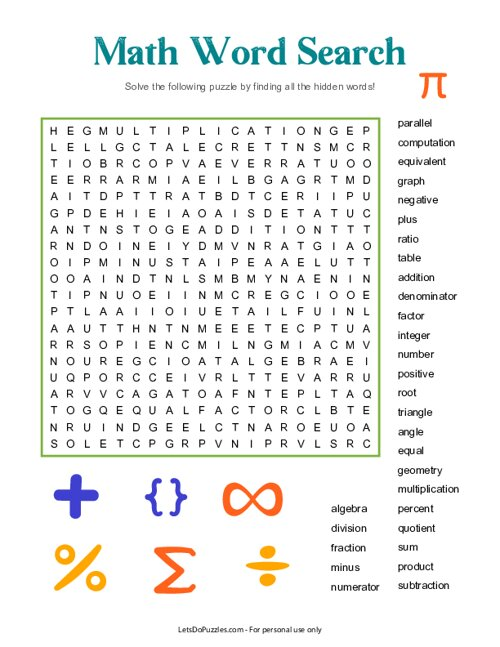# Math Terms Word Search

Download free printable Math Terms Word Search PDF to print on letter size paper.Dive into the world of math with this special Math Terms Word Search! Filled with interesting and challenging words, this word search puzzle is sure to engage the minds of your kids.

Not only will it provide hours of fun for them, but it will also help to spark their curiosity about math and other related topics. Download and print now so your kids can explore and get excited about concepts like algebra, geometry, subtraction, and more.

Words List: Addition, Algebra, Angle, Computation, Denominator, Division, Equal, Equivalent, Factor, Fraction, Geometry, Graph, Integer, Minus, Multiplication, Negative, Number, Numerator, Parallel, Percent, Plus, Positive, Product, Quotient, Ratio, Root, Subtraction, Sum, Table, Triangle

Math Terms Word Search